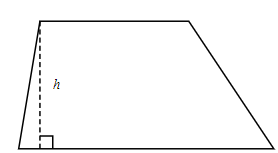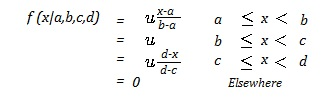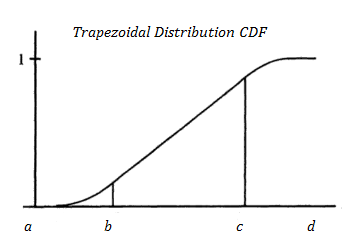# Trapezoidal Distribution: Definition, PDF, CFD

Probability Distributions > Trapezoidal Distribution

## What is a Trapezoidal Distribution?A trapezoid.Trapezoidal distributions are in the shape of a trapezoid— a quadrilateral with two parallel and two non-parallel sides. They tend to be a good fit for data that shows fairly rapid growth, a leveling out period, and then fairly rapid decay. All three stages are linear functions: The first stage is increasing with a positive slope; The leveling out period is constant and the final stage is decreasing with a negative slope.

Van Dorp & Kotz define the parameters of the distribution as:

• a = minimum value for the random variable,
• b = lower mode (where the constant stage starts),
• c = upper mode (where the constant stage ends),
• d = maximum value for the random variable,
• m = growth rate for the period between a and b,
• n = decay rate for the period between c and d,
• α = boundary ratio, fx(b)/fx(c) which Kotz & Van Dorp define as “the relative likelihood of capabilities at stage [a,b] and the beginning of the decay stage [c, d].”

Note that the mode of this distribution is not unique; it can take on any value between the lower mode c and the upper mode d.

## PDF of the Trapezoidal Distribution

The Probability Density Function (PDF) for the trapezoidal distribution (From Dorp & Kotz, 2003) is:Where:

• μ = 2/(d + c – b – a)-1
• a ≤ b ≤ c ≤ d

## CDFThe trapezoidal cumulative distribution function is 0 for x < a and 1 for x ≥ d. Otherwise, it is linear between b and c, and quadratic for a → b and c → d:## Similar Distributions

• The uniform distribution is a special case of the trapezoidal distribution; It does not have a growth or decay stage so a (the minimum) = c (the lower mode) and d (the maximum) = b (the upper mode).
• The triangular distribution is also a special case of the trapezoidal; It is missing the constant stage so b (the lower mode) = c (the upper mode).

References:
Kotz, S. & Dorp. R. (2004). Beyond Beta: Other Continuous Families of Distributions with Bounded Support and Applications. World Scientific.
Van Dorp, J et. al,. (2004). An Elicitation Procedure for the Generalized Trapezoidal Distribution with a Uniform Central Stage. Decision Analysis, 4(3):156–166, September 2007.
Van Dorp, J. & Kotz, S.(2003) Generalized Trapezoidal Distributions. Metrika, Vol. 58, Issue 1, July.

CITE THIS AS:
Stephanie Glen. "Trapezoidal Distribution: Definition, PDF, CFD" From StatisticsHowTo.com: Elementary Statistics for the rest of us! https://www.statisticshowto.com/trapezoidal-distribution/